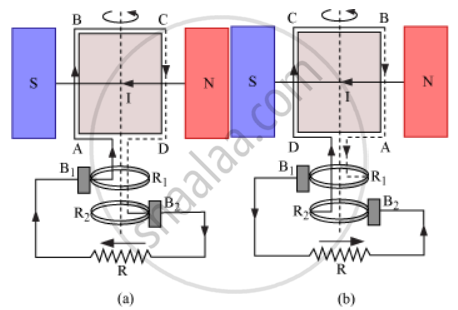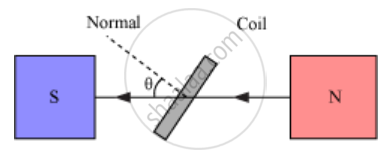# State the Principle of an Ac Generator and Explain Its Working with the Help of a Labelled Diagram. Obtain the Expression for the Emf Induced in a Coil. - Physics

State the principle of an ac generator and explain its working with the help of a labelled diagram. Obtain the expression for the emf induced in a coil. having N turns each of cross-sectional area A, rotating with a constant angular speed 'ω' in a magnetic field vecB directed perpendicular to the axis of rotation.

#### Solution

Principle - Based on the phenomenon of electromagnetic induction

ConstructionMain parts of an ac generator:

Armature − Rectangular coil ABCD

Filed Magnets − Two pole pieces of a strong electromagnet

Slip Rings − The ends of coil ABCD are connected to two hollow metallic rings R1 and R2

Brushes − B1 and B2 are two flexible metal plates or carbon rods. They are fixed and are kept in tight contact with R1 and R2 respectively.

Theory and Working − As the armature coil is rotated in the magnetic field, angle θ between the field and normal to the coil changes continuously. Therefore, magnetic flux linked with the coil changes. An emf is induced in the coil. According to Fleming’s right-hand rule, current induced in AB is from A to B and it is from C to D in the CD. In the external circuit, current flows from B2 to B1

To calculate the magnitude of emf induced:

Suppose

A → Area of each turn of the coil

N → Number of turns in the coil

vecB→ Strength of magnetic field

θ → Angle which normal to the coil makes with vecB at any instant t∴ Magnetic flux linked with the coil in this position:

phi = N(vecB.vecA) = NBA cosθ= NBA cosωt …(i)

Where ‘ω’ is angular velocity of the coil

As the coil rotates, angle θ changes. Therefore, magnetic flux Φ linked with the coil changes and hence, an emf is induced in the coil. At this instant t, if e is the emf induced in the coil, then

e = -(dtheta)/(dt) = - d/(dt) (NAB cos omegat)

= -NAB d/(dt) (cos omegat)

=-NAB(-sin omegat)omega

∴ e = NAB ω sinωt

Concept: AC Generator
Is there an error in this question or solution?